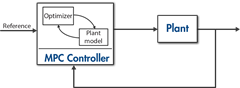# Get Started with Model Predictive Control Toolbox

Design and simulate model predictive controllers

Model Predictive Control Toolbox™ provides functions, an app, Simulink® blocks, and reference examples for developing model predictive control (MPC). For linear problems, the toolbox supports the design of implicit, explicit, adaptive, and gain-scheduled MPC. For nonlinear problems, you can implement single- and multi-stage nonlinear MPC. The toolbox provides deployable optimization solvers and also enables you to use a custom solver.

You can evaluate controller performance in MATLAB® and Simulink by running closed-loop simulations. For automated driving, you can also use the provided MISRA C™- and ISO 26262-compliant blocks and examples to quickly get started with lane keep assist, path planning, path following, and adaptive cruise control applications.

The toolbox supports C and CUDA® code and IEC 61131-3 Structured Text generation.

## Tutorials

• What is Model Predictive Control?

Introduction to MPC main concepts.

• MPC Signal Types

Plant inputs are independent variables that affect the plant, and plant outputs are dependent variables that you want to control or monitor.

• MPC Prediction Models

Model predictive controllers use plant, disturbance, and noise models for prediction and state estimation.

• Controller State Estimation

MPC controllers use their current state as the basis for predictions. In general, the controller states are unmeasured and must be estimated.

• Optimization Problem

Model predictive controllers compute optimal manipulated variable control moves by solving a quadratic program at each control interval.

• QP Solvers

The model predictive controller QP solvers convert an MPC optimization problem to a general form quadratic programming problem.

## VideosWhy Use MPC?
Understand the benefits of using model predictive control.What Is MPC?
Understand the working principles of model predictive control.MPC Design Parameters
Understand common MPC design parameters, such as sample time, horizons, tuning weights, and constraints.